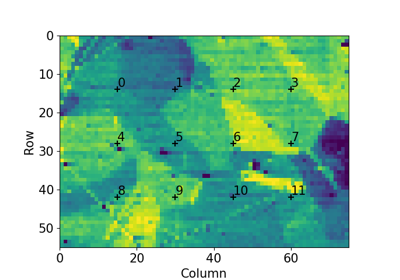# plot_pattern_positions_in_map#

kikuchipy.draw.plot_pattern_positions_in_map(rc: ndarray, roi_shape: tuple, roi_origin: tuple = (0, 0), area_shape: = None, roi_image: = None, area_image: = None, axis: = None, return_figure: bool = False, color: = 'k') [source]#

Plot pattern positions in a 2D map within a region of interest (ROI), the ROI potentially within a larger area.

Parameters:
rc

Position coordinates (row, column) in an array of shape (n, 2). If `area_shape` is passed, coordinates are assumed to be given with respect to the area origin, and so if `roi_origin` is passed, the origin is subtracted from the coordinates.

roi_shape

Shape of the ROI as (n rows, n columns).

roi_origin

Origin (row, column) of the ROI with respect to the area. If this and `area_shape` is passed, the origin is subtracted from the `rc` coordinates.

area_shape

Shape of the area including the ROI as (n rows, n columns). If this and `roi_origin` is passed, the origin is subtracted from the `rc` coordinates.

roi_image

Image to plot within the ROI, of the same aspect ratio.

area_image

Image to plot within the area, of the same aspect ratio.

axis

Existing Matplotlib axis to add the positions to. If not passed, a new figure will be created. If passed, only the coordinate markers and labels are added to the axis. E.g. `roi_image` or `area_image` will not be used.

return_figure

Whether to return the created figure. Default is `False`.

color

Color of position markers and labels. Default is `k`. Must be a valid Matplotlib color identifier.

Returns:
`fig`

Created figure, returned if `return_figure=True`.

## Examples using `plot_pattern_positions_in_map`#Extract patterns from a grid

Extract patterns from a grid